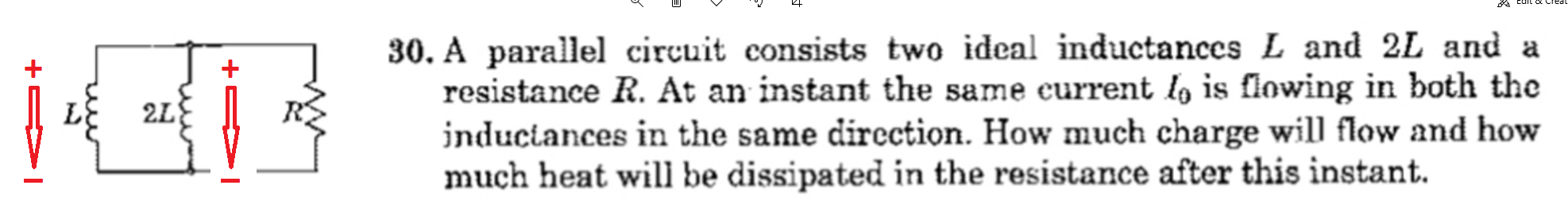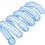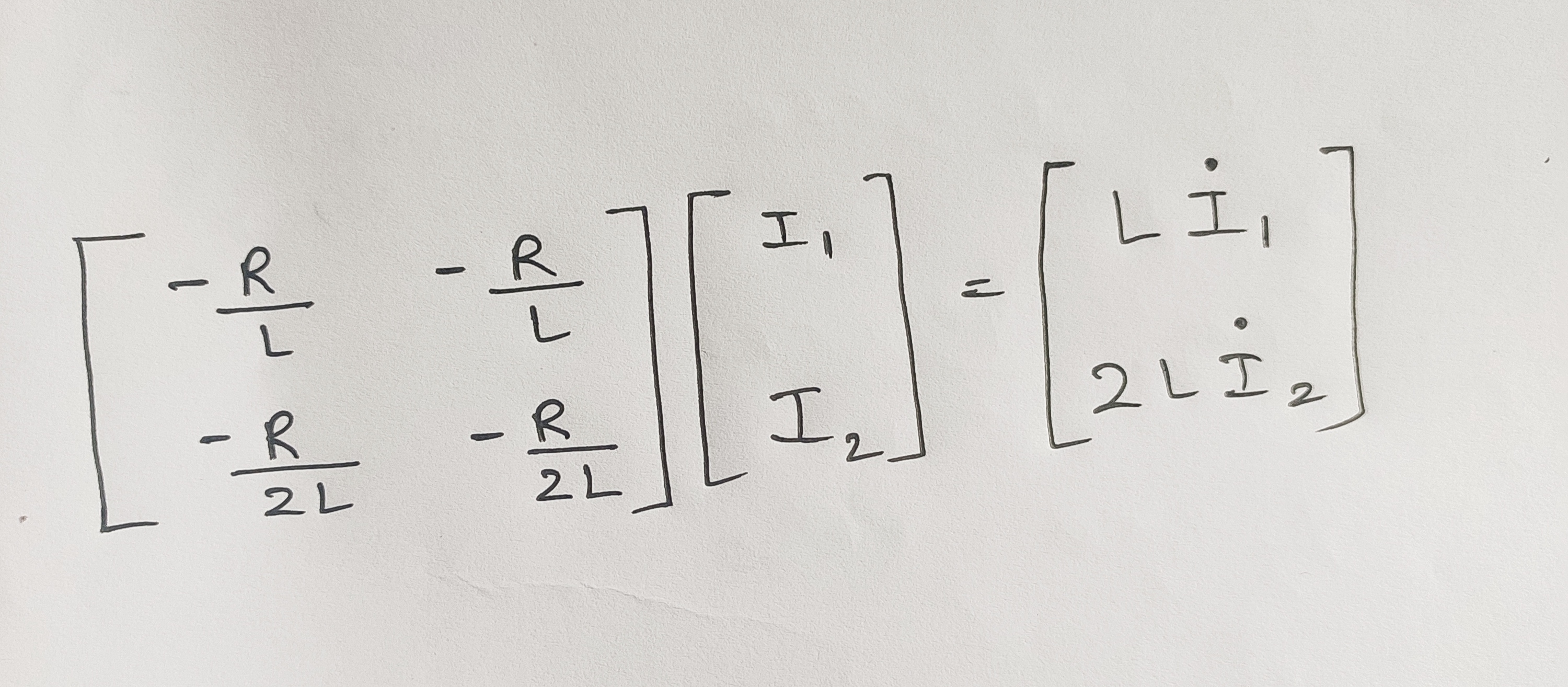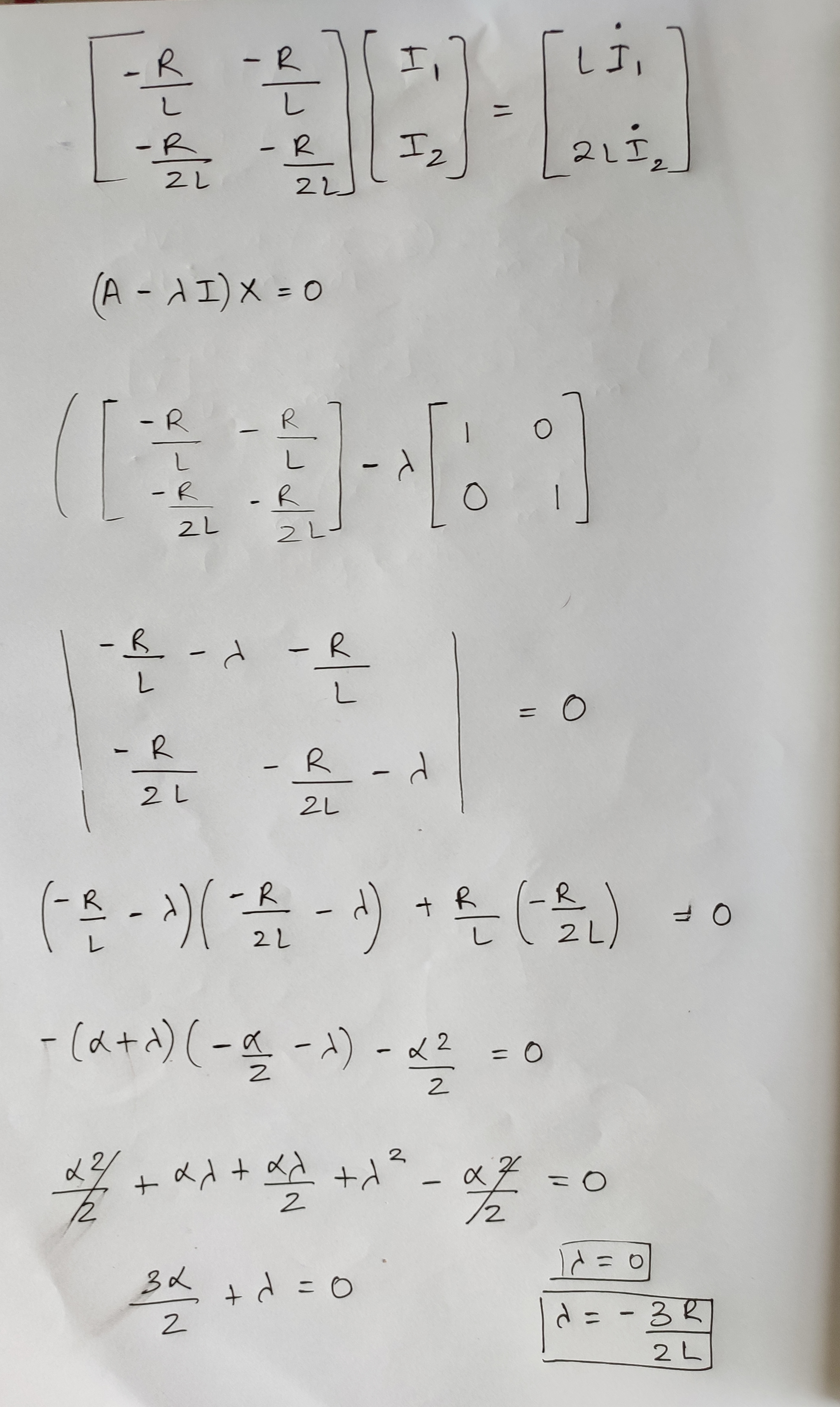# RL Diff Eq Exercise

Here is a problem sent by NeerajLet the current in the left inductor be $I_{L1}$ and let the current in the right inductor be $I_{L2}$.

Recall that the voltage across an inductor is equal to its inductance multiplied by the time derivative of its current. The equations governing the circuit are:

$-(I_{L1} + I_{L2})R = L \dot{I}_{L1} \\ -(I_{L1} + I_{L2})R = 2L \dot{I}_{L2}$

The system matrix is:

$\begin{bmatrix} - \frac{R}{L} & - \frac{R}{L} \\ - \frac{R}{2 L} & - \frac{R}{2 L} \end{bmatrix}$

The eigenvalues for this system are $(-\frac{3R}{2L}, 0)$, and the associated eigenvectors are $\begin{bmatrix} 2 \\ 1 \end{bmatrix}$ and $\begin{bmatrix} -1 \\ 1 \end{bmatrix}$. I used Wolfram alpha to find these.

The currents are therefore:

$\begin{bmatrix} I_{L1} \\ I_{L2} \end{bmatrix} = c_1 \begin{bmatrix} 2 \\ 1 \end{bmatrix} e^{-3R t / 2L} + c_2 \begin{bmatrix} -1 \\ 1 \end{bmatrix} e^{0 t }$

To solve for the constants, apply the initial conditions from time $t = 0$:

$\begin{bmatrix} I_0 \\ I_0 \end{bmatrix} = c_1 \begin{bmatrix} 2 \\ 1 \end{bmatrix} + c_2 \begin{bmatrix} -1 \\ 1 \end{bmatrix}$

This results in $(c_1, c_2) = ( \frac{2}{3} I_0, \frac{1}{3} I_0 )$.

The equations for the currents are then:

$I_{L1} = \frac{4}{3} I_0 e^{-3R t / 2L} - \frac{1}{3} I_0 \\ I_{L2} = \frac{2}{3} I_0 e^{-3R t / 2L} + \frac{1}{3} I_0 \$/extract_itex] Interestingly, the inductors don't spend all of their energy as heat lost to the resistor. At $t = \infty$, there is a circulating current of magnitude $\frac{1}{3} I_0$ that only flows in a loop through the inductors. The resistor current is: $I_R = -(I_{L1} + I_{L2}) = - 2 I_0 e^{-3R t / 2L}$ From here, one can calculate the heat and charge as follows: $E_h = \int_0^\infty I^2_R R \, dt \\ Q = \int_0^\infty I_R \, dt$Note by Steven Chase 11 months ago This discussion board is a place to discuss our Daily Challenges and the math and science related to those challenges. Explanations are more than just a solution — they should explain the steps and thinking strategies that you used to obtain the solution. Comments should further the discussion of math and science. When posting on Brilliant: • Use the emojis to react to an explanation, whether you're congratulating a job well done , or just really confused . • Ask specific questions about the challenge or the steps in somebody's explanation. Well-posed questions can add a lot to the discussion, but posting "I don't understand!" doesn't help anyone. • Try to contribute something new to the discussion, whether it is an extension, generalization or other idea related to the challenge. • Stay on topic — we're all here to learn more about math and science, not to hear about your favorite get-rich-quick scheme or current world events. MarkdownAppears as *italics* or _italics_ italics **bold** or __bold__ bold - bulleted- list • bulleted • list 1. numbered2. list 1. numbered 2. list Note: you must add a full line of space before and after lists for them to show up correctly paragraph 1paragraph 2 paragraph 1 paragraph 2 [example link](https://brilliant.org)example link > This is a quote This is a quote  # I indented these lines # 4 spaces, and now they show # up as a code block. print "hello world" # I indented these lines # 4 spaces, and now they show # up as a code block. print "hello world" MathAppears as Remember to wrap math in $$ ... $$ or \[ ... $ to ensure proper formatting.
2 \times 3 $2 \times 3$
2^{34} $2^{34}$
a_{i-1} $a_{i-1}$
\frac{2}{3} $\frac{2}{3}$
\sqrt{2} $\sqrt{2}$
\sum_{i=1}^3 $\sum_{i=1}^3$
\sin \theta $\sin \theta$
\boxed{123} $\boxed{123}$

Sort by:

@Lil Doug Greetings. Here is problem 30

- 11 months ago

@Steven Chase Thank you so much.
BTW, what is the meaning of eigen and how to find eigenvalues?

- 10 months, 4 weeks ago

"Eigen" is the German word for "characteristic" or "own". Here is an article with more details

https://brilliant.org/wiki/eigenvalues-and-eigenvectors/

- 10 months, 4 weeks ago

You'll have to study Linear Algebra formally to know what this is. I assume you're familiar with matrices, at the most basic level. So, an eigenvalue is found basically when you multiply a matrix by a vector, and you get a scaled version of the vector.

You can find it using the equation below:

$A \vec{v} = \lambda \vec{v}$

Where $A$ is a matrix, and $\lambda$ is the Eigenvalue.

What you're doing is you're going to be solving the equation above, to find the eigenvalue, and the eigenvector (Usually there'll be more than one) that satisfies the equation.

You'll find it really useful in the future.

- 10 months, 4 weeks ago

@Krishna Karthik bro how to type matrix in Brilliant?

- 10 months, 4 weeks ago

Like this:

"\begin{bmatrix} 1 & 2 & 3 \ a & b & c \end{bmatrix}"

Renders as: $\begin{bmatrix} 1 & 2 & 3\\ a & b & c \end{bmatrix}$

- 10 months, 4 weeks ago

@Krishna Karthik \$\begin{bmatrix} 1 & 2 & 3 \ a & b & c \end{bmatrix}$

- 10 months, 4 weeks ago

Use two back slashes between the rows. On brilliant, it only comes as one for a weird reason.

- 10 months, 4 weeks ago

@Steven Chase it is written at an instant, the current is flowing $I_{0}$ ,
Why we are integrating it from 0 to $infty$ ?
Maybe it can 3 to $\infty$??? 4 to $\infty$ ?

- 10 months, 4 weeks ago

The beginning instant has been arbitrarily chosen as $t = 0$

- 10 months, 4 weeks ago

@Steven Chase isn't there any pure Anayltical way to solve this??

- 10 months, 4 weeks ago

Which part don't you like?

- 10 months, 4 weeks ago

@Steven Chase I like all part
But I want a Anayltical way of that solution of differential equation.

- 10 months, 4 weeks ago

You mean the calculation of the eigen-quantities? You could do that by hand if you wanted to.

- 10 months, 4 weeks ago

@Steven Chase yeah can you guide me

- 10 months, 4 weeks ago

I can post a note on the general process tomorrow

- 10 months, 4 weeks ago

@Steven Chase Hello.

- 10 months, 4 weeks ago

@Krishna Karthik Bro this is the whole matrix. Now guide me to eigen- 10 months, 4 weeks ago

To find the eigenvalues, solve the equation:

$\det(A-\lambda I) = 0$

I want you to solve the equation resulting yourself to find out how to do this. The answer is already above. You need to learn how to solve for eigenvectors and eigenvalues.

We get a quadratic equation; solving this quadratic equation, we get the associated eigenvectors and eigenvalues. And since it's quadratic, there will be two eigenvectors.

- 10 months, 4 weeks ago

@Krishna Karthik bro what is det?

- 10 months, 4 weeks ago

Determinant of a matrix. It's almost like finding the volume from the paralleled of the matrix vectors. Or the area, in this case.

- 10 months, 4 weeks ago

@Krishna Karthik and what is $I$

- 10 months, 4 weeks ago

Identity matrix in 2 dimensions: $\begin{bmatrix}1 & 0 \\ 0 &1 \end{bmatrix}$

This is not to be confused with current.

- 10 months, 4 weeks ago

@Krishna Karthik Ok thanks bro. I am solving it right now.
I will show my solution within 10 min.

- 10 months, 4 weeks ago

Nice. Keep it going bro :)

- 10 months, 4 weeks ago

@Krishna Karthik here it isThanks

- 10 months, 4 weeks ago

Perfect bro. Well done :)

- 10 months, 4 weeks ago

Btw, I just want to add, an eigenvalue of 0 is never counted, because 0 is always a solution. You learnt this pretty quickly, I think. Good job.

- 10 months, 4 weeks ago

Also, watch this video guiding how to solve a system of differential equations. You need Linear Algebra knowledge for it:

- 10 months, 4 weeks ago

@Steven Chase Help me in the 35th problem.

- 10 months, 4 weeks ago

That's kind of a nice problem. I wouldn't mind posting that as a problem in the E and M section. And then we could have people post solutions to it (my own included). Or if you know the answer, you could post it.

- 10 months, 4 weeks ago

@Steven Chase I didn't understand what you want to say.
You can do anything what you want to do, at the end I want Anayltical solution.
By the see my new note please.

- 10 months, 4 weeks ago

@Steven Chase ok I am. Posting that problem right now.

- 10 months, 4 weeks ago

@Steven Chase it is up now.

- 10 months, 4 weeks ago